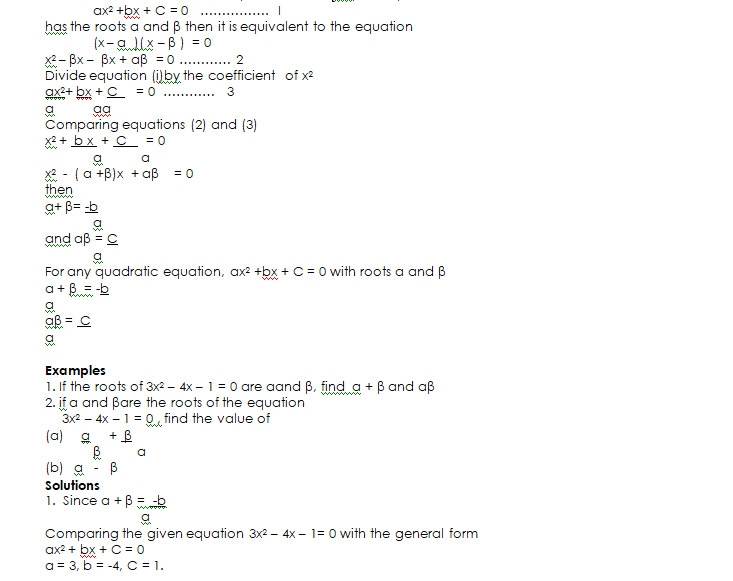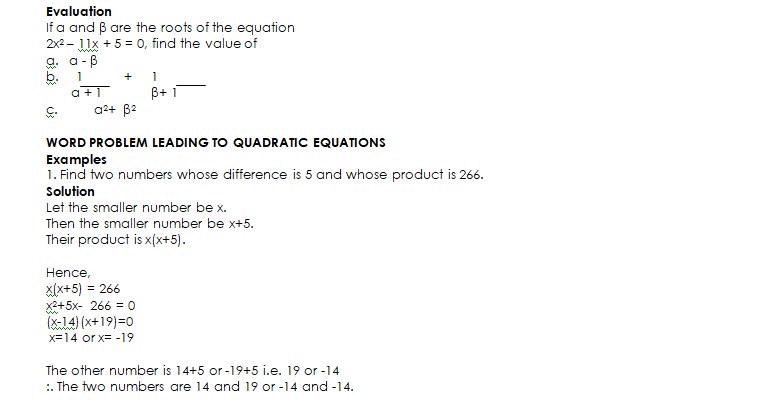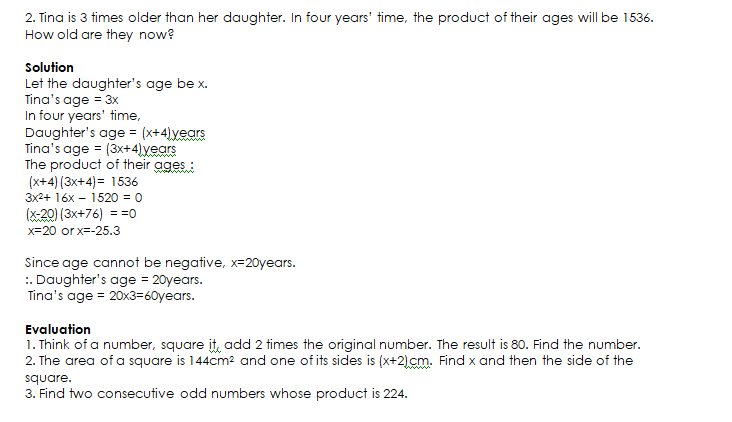Welcome to class!

In today’s class, we will be talking about the quadratic equation. Enjoy the class!#### CONSTRUCTION OF QUADRATIC EQUATIONS FROM SUM AND PRODUCT OF ROOTS

We can find the sum and product of the roots directly from the coefficient in the equation. It is usual to call the roots of the equation α and β  If the equation###### GENERAL EVALUATION
1. The area of a rectangle is 60cm2. The length is 11cm more than the width. Find the width.
2. A man is 37years old and his child is 8. How many years ago was the product of their ages 96?
3. If α and β are the roots of the equation 2x2 – 9x+4=0, find
4. a) α + β (b) αβ (c) α – β   (d)  αβ/ α + β

In our next class, we will be talking about Simultaneous Equations.  We hope you enjoyed the class.

Should you have any further question, feel free to ask in the comment section below and trust us to respond as soon as possible.

How Can We Make ClassNotesNG Better - CLICK to Tell Us💃